# ICSE Class 9 Maths Important Questions

ICSE class 9 is a significant period in a student’s life as class 9 is where the base-level preparations for class 10 begin. Hence, the subject specialists at BYJU’S prepare ICSE Class 9 Maths important questions based on the latest ICSE Class 9 Maths syllabus prescribed by the Board. The ICSE Class 9 Important Questions adorn the students with a brief insight regarding the essential topics, important and sure-shot question types etc. By following ICSE Class 9 Maths important questions, students can evaluate their level of understanding and knowledge of the subject, in turn helping them to augment their self-confidence.

The important questions of Maths will help them to reveal areas on which they need to work on after solving each and every important question of Class 9 Maths. Students of ICSE Class 9 can easily make an about the upcoming exam question by solving these important questions. It covers all the important concepts and formulas that need to be thorough to score good marks. There is a chance that these important questions of Class 9 Maths can be asked in the board exam.

For the benefit of the students, we have provided below the ICSE Class 9 Important Questions of Maths so that they become experts in solving any type of questions asked during the exam.

1. Rationalise the denominator: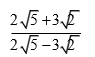2. Two circles touch externally. The sum of their areas is 130πsq. cm and the distance between their centres is 14 cm. Find the radii of the circles.
3. A car manufacturing company increases the production of a particular type of car in 2 years from 2,16,000 to 3,11,040. Find the annual rate of growth of production.
4. In the following figure, ABCD is a rhombus, and DCFE is a square.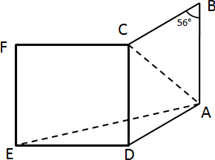If ∠ABC = 56°, find(i) ∠DAE (ii) ∠FEA (iii) ∠EAC (iv) ∠AEC
5. Solve using cross-multiplication:
1. 4x + 3y = 17
2. 3x – 4y + 6 = 0
6. If 2 sin θ – 2 = 0 and 0° ≤ θ ≤ 90°, find the value of θ.
7. AB and AC are two chords of a circle of radius r such that AB = 2AC. If p and q are the distances of AB and AC from the centre, then prove that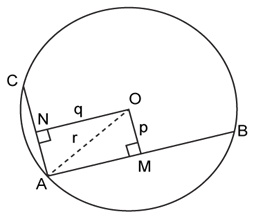8. Find the point on the x-axis which is equidistant from the points A(−2, 5) and B(2,−3)
9. Construct a regular hexagon of side 2.5 cm.
10. If x + y – z = 4 and x2 + y2 + z2 = 30, then find the value of xy – yz – zx.
11. Use the adjoining figure to find,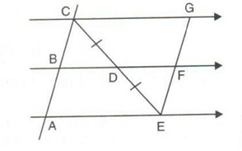1. BC, if AB = 8.6 cm
2. GE, if FE = 6 cm
3. AE, if BD = 3.6 cm
4. DF, if CG = 15 cm
12. Prove that the perimeter of a triangle is greater than the sum of its three medians.
13. If the mean of five observations x, x + 2, x + 4, x + 6, x + 8 is 13, find the value of x, and hence, find the mean of the last three observations.
14. If the mean of five observations x, x + 2, x + 4, x + 6, x + 8 is 13, find the value of x, and hence, find the mean of the last three observations.
15. A cuboidal water tank is 6 m long, 5 m wide, and 4.5 m deep. How many litres of water can it hold? (Given 1 m3 = 1000 litres)
16. If P is the centre of the circle with a radius of 6.7 cm, d(P, Q) = 7.6 cm, d(P, R) = 5.7 cm, find the positions of points R and Q.
17. Solve the following simultaneous equations using the graphical method: x + y = 8; x – y = 2
18. The line segments joining the mid-points M and N of parallel sides AB and DC, respectively, of a trapezium ABCD is perpendicular to both sides AB and DC. Prove that AD = BC.
19. In the given figure, AB ∥ DE and BD ∥ FG such that ∠ABC = 50° and ∠FGH = 120°. Find the values of x and y.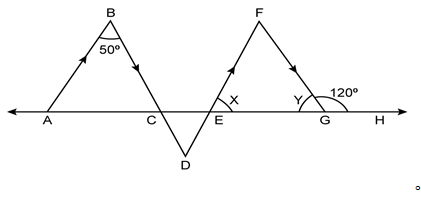20. Each equal angle of an isosceles triangle is less than the third angle by 15 degrees. Find the angles.

### Benefits of solving ICSE Class 9 Maths Important Questions

• It gives confidence to the students to solve the question paper correctly.
• It improves their performance and helps them to work on their weak points.
• It is a valuable resource for revision purposes in a quick span of time.
• ICSE Important Questions covers all the crucial concepts and formulas.